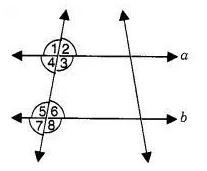# State the property that is used each of the following statements?

State the property that is used each of the following statements ?

1. If a || b, then ∠ 1 = ∠ 5.
2. If ∠ 4 = ∠ 6, then a || b.
3. If ∠ 4 + ∠ 5 = 180°, then a || b.